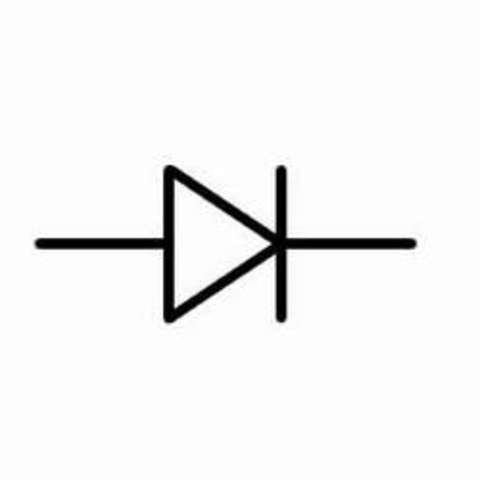## Tutorials / Beginning Electronics

### Beginning Electronics*Under Construction*

Before we get into electronics, we should start with electricity. Why should you learn about electricity? Because it is dangerous and it can hurt you but also awesome... so you should find out what you shouldn't do before you go exploring the wonderful world of electronics. I will try to devise a set of 10 steps that you can (safely) follow to get you started exploring in the right direction.

Electricity is simply heat that has been harnessed into a conductor (like a copper wire) - if your body becomes the conductor, you can get burned by this heat as it has the potential to travel through your body. To avoid getting shocked, you should know how to determine if an electrical signal has the potential to harm you and how to proceed safely if it does. I know you don't want to read a book, so I'll try to keep it short.

There are 4 main parts of electriciy that should be discussed prior to experimenting. Voltage, Amperage, Wattage, and Resistance (there are others, but not now). These 4 parts make up a basic electrical signal: the voltage can be thought of as the potential force of the signal - its level will determine if it is dangerous. The amperage represents the amount of electrical current that flows past a given point each second - corresponds to the power of the signal. The wattage is a combination of the amperage and voltage and represents a total measure of heat that will be produced by the circuit. Resistance is the slowing of electrical current flow due to poor conductivity - in general, when electricity encounters resistance it turns into heat, so knowing how to calculate the resistance in a circuit can be very useful. Which leads us to Ohm's Law (read about Ohm):

Voltage = Current x Resistance

Wattage = Current x Voltage

Wattage = Resistance x Current2

... more coming soonish